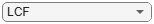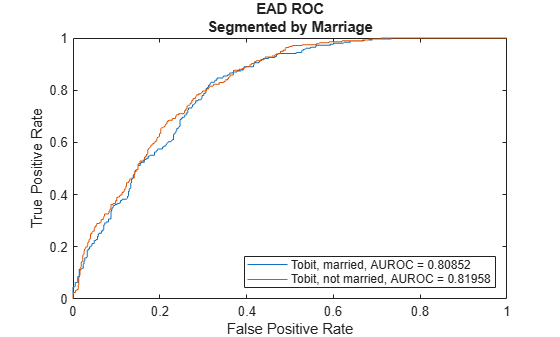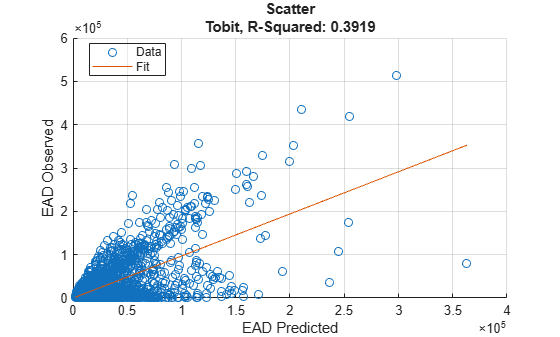modelAccuracy

Compute R-square, RMSE, correlation, and sample mean error of predicted and observed EADs

Description

example

AccMeasure = modelAccuracy(eadModel,data) computes the R-square, root mean square error (RMSE), correlation, and sample mean error of observed vs. predicted exposure at default (EAD) data. modelAccuracy supports comparison against a reference model and also supports different correlation types. By default, modelAccuracy computes the metrics in the EAD scale. You can use the ModelLevel name-value pair argument to compute metrics using the underlying model's transformed scale.

example

[AccMeasure,AccData] = modelAccuracy(___,Name,Value) specifies options using one or more name-value pair arguments in addition to the input arguments in the previous syntax.

Examples

collapse all

This example shows how to use fitEADModel to create a Tobit model and then use modelAccuracy to compute the R-Square, RMSE, correlation, and sample mean error of predicted and observed EAD.

ans=8×6 table
UtilizationRate    Age     Marriage        Limit         Drawn          EAD
_______________    ___    ___________    __________    __________    __________

0.24359        25     not married         44776         10907         44740
0.96946        44     not married    2.1405e+05    2.0751e+05         40678
0        40     married        1.6581e+05             0    1.6567e+05
0.53242        38     not married    1.7375e+05         92506        1593.5
0.2583        30     not married         26258        6782.5        54.175
0.17039        54     married        1.7357e+05         29575        576.69
0.18586        27     not married         19590          3641        998.49
0.85372        42     not married    2.0712e+05    1.7682e+05    1.6454e+05

rng('default');
c = cvpartition(NumObs,'HoldOut',0.4);
TrainingInd = training(c);
TestInd = test(c);

Select Model Type

Select a model type for Tobit or Regression.

ModelType ="Tobit";

Select Conversion Measure

Select a conversion measure for the EAD response values.

ConversionMeasure ="LCF";

Tobit with properties:

CensoringSide: "both"
LeftLimit: 0
RightLimit: 1
ModelID: "Tobit"
Description: ""
UnderlyingModel: [1x1 risk.internal.credit.TobitModel]
PredictorVars: ["UtilizationRate"    "Age"    "Marriage"]
LimitVar: "Limit"
DrawnVar: "Drawn"
ConversionMeasure: "lcf"

Display the underlying model. The underlying model's response variable is the transformation of the EAD response data. Use the 'LimitVar' and 'DrwanVar' name-value arguments to modify the transformation.

Tobit regression model:
Y* ~ 1 + UtilizationRate + Age + Marriage

Estimated coefficients:
Estimate         SE         tStat       pValue
__________    __________    ________    ________

(Intercept)                0.22735      0.025045      9.0776           0
UtilizationRate            0.47364      0.016536      28.643           0
Age                     -0.0013929    0.00061488     -2.2654    0.023537
Marriage_not married     -0.006888      0.012113    -0.56863     0.56964
(Sigma)                    0.36419     0.0038746      93.995           0

Number of observations: 4378
Number of left-censored observations: 0
Number of uncensored observations: 4377
Number of right-censored observations: 1
Log-likelihood: -1791.06

EAD prediction operates on the underlying compact statistical model and then transforms the predicted values back to the EAD scale. You can specify the predict function with different options for the 'ModelLevel' name-value argument.

For model validation, use modelDiscrimination, modelDiscriminationPlot, modelAccuracy, and modelAccuracyPlot.

Use modelDiscrimination and then modelDiscriminationPlot to plot the ROC curve.

ModelLevel ="ead";Use modelAccuracy and then modelAccuracyPlot to show a scatter plot of the predictions.

YData ="Observed";

AccMeasure1=1×4 table
RSquared    RMSE     Correlation    SampleMeanError
________    _____    ___________    _______________

Tobit    0.39127     42545      0.62552          -1713.1

AccData1=1751×3 table
Observed     Predicted_Tobit    Residuals_Tobit
__________    _______________    _______________

44740           15177              29563
54.175          8900.3            -8846.1
987.39           13430             -12443
9606.4          7422.4               2184
83.809           27852             -27768
73538           46229              27309
96.949          5582.8            -5485.9
873.21          4527.1            -3653.9
328.35          6079.8            -5751.5
55237           28295              26942
30359           19177              11182
39211           28753              10457
2.0885e+05      1.0725e+05          1.016e+05
1921.7           20132             -18210
15230          5526.4             9703.5
20063          9501.2              10562
⋮Input Arguments

collapse all

Loss given default model, specified as a previously created Regression or Tobit object using fitEADModel.

Data Types: object

Data, specified as a NumRows-by-NumCols table with predictor and response values. The variable names and data types must be consistent with the underlying model.

Data Types: table

Name-Value Arguments

Specify optional comma-separated pairs of Name,Value arguments. Name is the argument name and Value is the corresponding value. Name must appear inside quotes. You can specify several name and value pair arguments in any order as Name1,Value1,...,NameN,ValueN.

Correlation type, specified as the comma-separated pair consisting of 'CorrelationType' and a character vector or string.

Data Types: char | string

Data set identifier, specified as the comma-separated pair consisting of 'DataID' and a character vector or string. The DataID is included in the output for reporting purposes.

Data Types: char | string

Model level, specified as the comma-separated pair consisting of 'ModelLevel' and a character vector or string.

Note

Regression models support all three model levels, but a Tobit model supports model levels only for 'ead' and 'conversionMeasure'.

Data Types: char | string

EAD values predicted for data by the reference model, specified as the comma-separated pair consisting of 'ReferenceEAD' and a NumRows-by-1 numeric vector. The modelAccuracy output information is reported for both the eadModel object and the reference model.

Data Types: double

Identifier for the reference model, specified as the comma-separated pair consisting of 'ReferenceID' and a character vector or string. 'ReferenceID' is used in the modelAccuracy output for reporting purposes.

Data Types: char | string

Output Arguments

collapse all

Accuracy measure, returned as a table with columns 'RSquared', 'RMSE', 'Correlation', and 'SampleMeanError'. AccMeasure has one row if only the eadModel accuracy is measured and it has two rows if reference model information is given. The row names of AccMeasure report the model ID and data ID (if provided).

Accuracy data, returned as a table with observed EAD values, predicted EAD values, and residuals (observed minus predicted). Additional columns for predicted and residual values are included for the reference model, if provided. The ModelID and ReferenceID labels are appended in the column names.

collapse all

Model Accuracy

Model accuracy measures the accuracy of the predicted probability of EAD values using different metrics.

• R-squared — To compute the R-squared metric, modelAccuracy fits a linear regression of the observed EAD values against the predicted EAD values:

$EA{D}_{obs}=a+b\ast EA{D}_{pred}+\epsilon$

The R-square of this regression is reported. For more information, see Coefficient of Determination (R-Squared).

• RMSE — To compute the root mean square error (RMSE), modelAccuracy uses the following formula where N is the number of observations:

$RMSE=\sqrt{\frac{1}{N}{\sum }_{i=1}^{N}\left(EA{D}_{i}^{obs}-EA{D}_{i}^{pred}{\right)}^{2}}$

• Correlation — This metric is the correlation between the observed and predicted EAD:

$corr\left(EA{D}_{obs},EA{D}_{pred}\right)$

• Sample mean error — This metric is the difference between the mean observed EAD and the mean predicted EAD or, equivalently, the mean of the residuals:

$SampleMeanError=\frac{1}{N}{\sum }_{i=1}^{N}\left(EA{D}_{i}^{obs}-EA{D}_{i}^{pred}\right)$

 Baesens, Bart, Daniel Roesch, and Harald Scheule. Credit Risk Analytics: Measurement Techniques, Applications, and Examples in SAS. Wiley, 2016.

 Bellini, Tiziano. IFRS 9 and CECL Credit Risk Modelling and Validation: A Practical Guide with Examples Worked in R and SAS. San Diego, CA: Elsevier, 2019.

 Brown, Iain. Developing Credit Risk Models Using SAS Enterprise Miner and SAS/STAT: Theory and Applications. SAS Institute, 2014.

 Roesch, Daniel and Harald Scheule. Deep Credit Risk. Independently published, 2020.